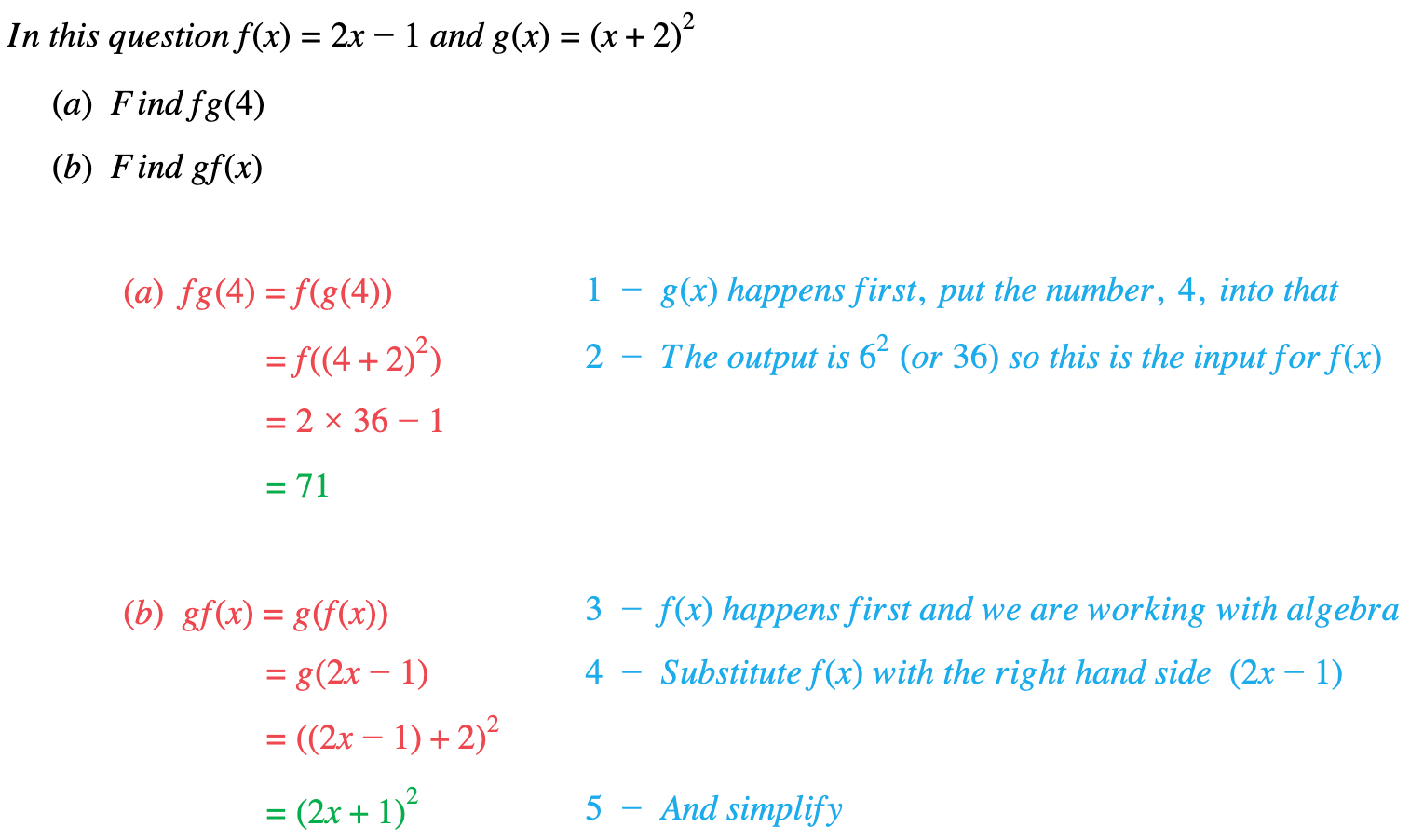# Edexcel IGCSE Maths 复习笔记 3.2.2 Compound Functions

Edexcel IGCSE Maths 复习笔记 3.2.2 Compound Functions

#### What is a compound function?

• A compound function is one function applied to the output of another function

#### What do compound functions look like?

• The notation you will see is:fg(x)
• it can be written as:f(g(x))and means “f applied to the output of g(x)” – ie. g(x) happens FIRST !

#### How does a compound function work?

• If you are putting a number into fg(x):
1. Put the number into g(x)
2. Put the output into f(x)eg. if f(x) = 2x + 1 and g(x) = 1/xthen fg(2) = f(½) =2 × ½ + 1 = 2and gf(2) = g(2 × 2 + 1) = g(5) = 1/5
• If you are using algebra:
1. For fg(x) put g(x) wherever you see x in f(x)
2. Substitute g(x) with the right hand side of g(x)=…
3. SIMPLIFY if necessaryeg. if f(x) = 2x + 1 and g(x) = 1 / xthen fg(x) = f(1/x) = 2 × 1 / x + 1 = 2 / x +1

and gf(x) = g(2 × x + 1) = g(2x + 1) = 1 / 2x+1

#### Exam Tip

Make sure you are applying the functions in the correct order.The letter nearest the bracket is the function applied first.

#### Worked Example